# The reaction of isotope-substituted hydrated iodide I(H182O)− with ozone: the reactive influence of the solvent water molecule†

Henrik B. Pedersen*a, Jonas Elmb, Christian H. Frederiksen a, Simon P. S. Jessen a, Ricky Teiwes a and Merete Bilde b
aDepartment of Physics and Astronomy, Aarhus University, DK-8000 Aarhus C, Denmark. E-mail: hbp@phys.au.dk; Tel: +45 8715 5653
bDepartment of Chemistry, Aarhus University, DK-8000 Aarhus C, Denmark

Received 15th June 2020 , Accepted 27th July 2020

First published on 20th August 2020

We report an investigation of the reaction of isotope-substituted hydrated iodide I(H182O) with ozone 16O3 to examine the involvement of the water molecules in the oxidation reactions that terminate with the formation of IO3. Experimentally, we studied the reaction in the gas phase as elementary reactions using a radio-frequency (RF) ion-trap combined with a quadrupole mass spectrometer (QMS). In approximately 1.2% of the reactions of I(H182O) and 16O3, the 18O atom is found to appear in iodine oxide anions, thus giving evidence for a close involvement of the water molecule in a non-negligible number of the reactions towards IO3. As a part of the experimental investigation, the reaction rate constant for the exchange reaction I(H182O) + H162O → I(H162O) + H182O at 300 K was found to be (1.3 ± 0.1) × 10−8 cm3 s−1. Quantum chemical calculations are exploited to establish the energetic difference between I(H182O) and I(H162O).

## 1 Introduction

Inorganic molecules containing iodine, in particular I2, IO, and HIO are observed both over the Earth's open oceans and over snow and sea ice,1–3 where they affect the atmospheric conditions through complex reaction cycles, e.g. leading to ozone destruction and new particle formation.2–4

Experiments5–8 and kinetic models8–10 strongly suggest that the release of HOI and I2 from aqueous solutions is initiated by the fundamental reaction of the iodide anion with ozone, i.e. I + O3. Thus, variations of this reaction can occur in the inter-facial air–water layer,6,8 in the aqueous bulk,9,11 inside sea-salt aerosols,10 or possibly in the gas phase.12,13 Several fundamental aspects of these reactions still remain to be investigated, and detailed accounts of the molecular reaction mechanisms in the various environments (surface, bulk, and gas phase) are challenging.12,14

Using an ion trap setup, we have previously investigated the oxidation of isolated bare iodide I ions by ozone in the gas phase12 and established that three sequential oxygen addition processes completely account for the observed reaction products, which terminate with the production of IO3, i.e.

 I− + O3 → IO− + O2

 IO− + O3 → IO2− + O2

 IO2− + O3 → IO3− + O2

A particularly important aspect of the I + O3 reaction in natural environments is the significance of associated water molecules (i.e. as solvent or through humidity) that can affect the actual processes both in terms of absolute reaction rate constants and reaction pathways. In a recent study,13 we have indeed demonstrated that when the iodide ion is associated with a single water molecule, i.e. I(H2O), the dominant reaction path for the reactions with O3 is markedly different from the sequential oxidation seen for bare iodide (I).12 Thus, the oxidation of the hydrated system I(H2O) proceeds mainly via the direct production of I and especially IO2, i.e.

 I(H2O)− + O3 → I− + neutrals

 → IO2−+ neutrals,
which is then further oxidized to IO3, in contrast to the direct production of IO seen for I.

In this paper, we use the same experimental setup as before12,13 to gain further information on the actual influence of the associated water molecule on the I(H2O) + O3 reaction by performing experiments with I(H182O) ions, i.e. where the oxygen atom of the water molecule is in the form of the heavy 18O isotope, while the oxygen atoms of the reacting ozone (O3) molecules are all of the naturally abundant 16O isotope. We demonstrate explicitly that a fraction of the observed oxidation products (especially the terminating IO3 product) is formed with an 18O atom originating from the initially associated water molecules. This observation suggests a deep mechanistic involvement (i.e. beyond the carrier of energy and momentum) of the water molecule in the actual reaction mechanism.

## 2 Experimental system

For the present experiment, we used the ion beam production, ion acceleration, ion mass selection, and electrostatic steering and focussing, as well as the ion–molecule reaction setup described previously.12,13 Briefly, ions were produced in a hollow cathode ion source with an inlet of iodine vapor sublimating from solid iodine crystals, N2 gas, and H182O vapor, extracted and accelerated to a kinetic energy of 3 kV, and mass-analyzed in a magnetic field. An example of ion currents obtained after mass selection is displayed in Fig. 1. While the atomic anion I dominated the spectrum with typical currents in excess of 30 nA, sufficient intensity (∼100 pA) for the present ion–molecule experiments was obtained for the hydrated anion. After mass selection, pulses of ions (either I or I(H182O)) were guided, decelerated, and trapped in the radio-frequency trap situated at a high voltage potential of ∼−3 kV.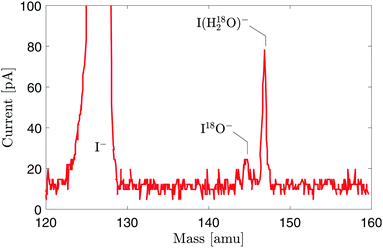Fig. 1 Spectrum of ion currents extracted from the hollow cathode ion source as a function of ion mass (magnetic field in a separator magnet) as measured behind the ion trap. For the spectrum shown, the ion source13 was operated with a discharge of approximately 4 mA at 450 V and an inlet of I2 vapor, N2 (3.5 mbar), and H182O (3.5 mbar).

The pressure in the ion trap chamber was sustained at 1–2 × 10−9 mbar without gas inlet. For the ion–molecule experiments, either water vapor (e.g. H162O) or a mixture of oxygen (O2) and ozone (O3)12 was leaked into the ion trap chamber raising the pressure in this region to a selected value in the range of 10−4–10−7 mbar. Thus, the inlet gas completely dominated the ion–molecule collisions in the trap, while its concentration was still sufficiently low to ensure that only binary collisions occured.

After a variable trapping time (2–100 ms in the present experiment), the trapped ions were extracted through a quadrupole mass spectrometer (QMS) and the amount of a particular product ion mass was registered with a channel electron multiplier (channeltron). To obtain a complete spectrum of product ion masses generated in the ion trap, the mass selection of the QMS was varied. Typically 100 experimental cycles of ion injection, trapping, and extraction were performed for each setting of the QMS.

The evolution of the product ion intensities as a function of the ion–molecule reaction time, IP(t), where P = I, IO, etc., was monitored by varying both the selected mass (QMS) and the trapping time in the RF-trap, while still spending 100 experimental cycles on each step. The two dimensional scanning was made by selecting a particular time step and then scanning through the selected masses. Additionally, before proceeding to the next trapping time, a reference signal IR was recorded for a selected product mass at a selected trapping time. Using the recorded reference signal, the measured intensities were corrected for the variation of the intensity of the primary ion beam throughout the total experimental period (several hours). Thus, the final experimental intensity obtained for a given product mass and trapping time was obtained as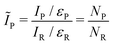(1)
where εP and εR represent the total detection efficiencies13 for fragments of types P and R.

## 3 Computational investigations

Geometry optimizations and calculations of harmonic vibrational frequencies were performed using the Gaussian 1615 program with the ωB97X-D16 density functional. To allow direct comparison to our previous studies we used the Gaussian 09 default values for convergence criteria and integral accuracy. The aug-cc-pVTZ basis set was used for oxygen/hydrogen atoms and the aug-cc-pVTZ-PP17 basis set was used for the iodine atom. A relativistic effective core potential was used to replace the 28-electron core of iodine.18 All thermochemical parameters were calculated at standard conditions (298.15 K and 1 atm.), using rigid-rotor and harmonic oscillator approximations. Calculations were performed for both the 16O and 18O isotopologues. The effect of anharmonicity was tested using the reported scaling factor of 0.95719 for the ωB97X-D/aug-cc-pVTZ level of theory and was found to have a negligible effect on the results.

In this paper, we are essentially interested in the isotope dependency of the following complex formation reaction

 I− + H2O → I(H2O)−, (2)
where the O-atom can be either 16O or 18O. For this reaction, the binding Gibbs free energy is given by
 ΔGbind = GI(H2O)− − GI− − GH2O, (3)
where the terms on the right hand side are the Gibbs free energies for the individual species. The binding Gibbs free energy can be decomposed as
 ΔG = ΔH − TΔS (4)
where ΔH is the binding enthalpy and ΔS is the binding entropy. A practical approach is to further partition eqn (4) in the following manner
 ΔG = ΔE + ΔGthermal (5)
where ΔE is the pure electronic binding energy and ΔGthermal collects all isotope- and thermally dependent contributions under equilibrium conditions. The partitioning in eqn (5) represents an explicit separation of the isotope-independent electronic energy surface from the thermal contribution to the Gibbs free energy.

When considering reactions involving different isotopologues under non-equilibrium conditions, as is the case under the present experimental situations (see Section 4.2), an essential number is the change in the zero-point-vibrational energy (ZPVE) associated with the particular reactions. The ZPVE for a particular molecule is given by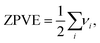(6)
where νi is the fundamental energy of the i-th vibrational mode of the molecule. With the described procedure of calculation, using the Gaussian 1615 program, we estimate a total accuracy of the ZPVE of ∼0.1 meV.

The change in vibrational frequencies between using different quantum chemical methods is to a large extent systematic. Thus, differences in ZPVE are not expected to be significantly influenced by using a higher level of theory. Anharmonicity usually leads to a lowering in the vibrational frequencies. To get an estimate of the effect of anharmonicity on the results, we used the vibrational scaling factor (0.957) for the ωB97X-D/aug-cc-pVTZ level of theory from the Computational Chemistry Comparison and Benchmark DataBase (CCCBDB).19 This changes the difference in ZPVE for the I(H182O) + H162O system and the I(H162O) + H182O system from 0.43 meV to 0.41 meV, and hence, anharmonicity is not expected to influence the presented results.

Table 1 shows the results of the calculations of isotope-dependent parameters (see eqn (5) and (6)) for the hydrated iodide (I(H162O) and I(H182O)) complexes as well as the isolated water molecules ((H162O) and (H182O)). For the water molecules, the vibrational frequencies correspond to the bend (ν0), the symmetric stretch (ν1), and the asymmetric stretch (ν2). For the hydrated iodides, the vibrational modes ν3ν5 correspond to the perturbed fundamental frequencies ν0ν2 of the water molecules.

Table 1 Harmonic vibrational frequencies, total zero-point vibrational energy (ZPVE), and thermal contribution to the Gibbs free energy (see eqn (5)) for hydrated iodide complexes and isolated water molecules. Note that the vibrational frequencies are given in units of cm−1
System ν 0 (cm−1) ν 1 (cm−1) ν 2 (cm−1) ν 3 (cm−1) ν 4 (cm−1) ν 5 (cm−1) ZPVE (meV) TΔS (meV) ΔGthermal (meV)
I(H162O) 129.1 240.8 581.2 1668.7 3650.5 3929.0 632.3 881.4 −109.5
I(H182O) 123.7 239.8 579.4 1661.9 3639.9 3916.2 629.9 885.6 −115.9
H162O 1634.1 3881.0 3988.2 589.1 582.5 109.4
H182O 1627.3 3872.8 3971.8 587.2 586.8 103.2

For the lowest vibrations of the I(H2O) complex, ν0 corresponds to the H2O rock, ν1 to the twist, and ν3 to the wag vibrational modes.

## 4 Experimental results

Fig. 2 shows the spectra of product ions obtained after injection of either bare iodide I or hydrated iodide I(H182O) in the presence of pure water vapor (H2O), oxygen (O2), or a mixture of oxygen and ozone (O3) at trapping times of 2.2 ms, 30 ms and 100.2 ms, respectively. The data shown in Fig. 2 have not been corrected for variations of the intensity of injected ions (I or I(H182O)), and the shown spectra are indicative of the resulting ion mass intensities present in the trap. A quantitative comparison of the intensities of the various masses are given later (see Fig. 4–6).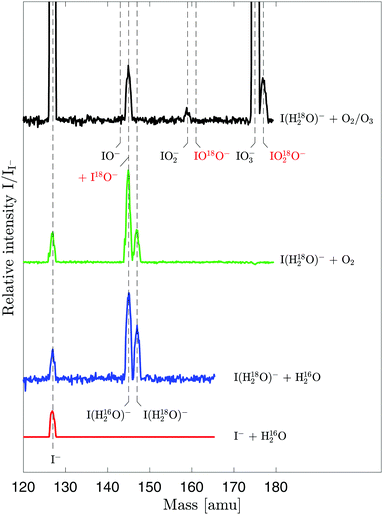Fig. 2 Mass spectra of trapped ions under different conditions of injected ions, gas inlets, and trapping times. For this display, the spectra have been normalized to the intensity of I− at the applied trapping time. The measurements with the inlet of water vapor were performed with a mean water pressure of pH2O = 1.4 × 10−6 mbar ([H2O] = 3.3 × 1010 cm−3 at 300 K) and a trapping time of 2.2 ms. The measurements with the inlet of O2 were done with a pressure of pO2 = 2.2 × 10−5 mbar ([O2] = 5.4 × 1011 cm−3 at 300 K) at 30 ms trapping time. The measurements with the inlet of O2/O3 were done with a total pressure pO2 + pO3 = 1.9 × 10−5 mbar and an ozone fraction of ∼4% ([O2] = 4.3 × 1011 cm−3 and [O3] = 1.8 × 1010 cm−3 at 300 K) at a late trapping time of 100.2 ms, where the intensity of the injected I(H182O−) was essentially gone.

Although our main goal was to gain insight into the reactions with ozone, we studied at first the reactions of trapped I and I(H182O) with water vapor, because the experiments with trapped I(H182O) ions will inherently be very sensitive to the presence of trace amounts of water (H162O) in the experimental systems, since the exchange of H182O with H162O is almost barrierless (a reaction barrier of 0.43 meV, see Table 1 and Section 4.2 below) and it is potentially associated with a significant reaction rate constant. While our chamber with the RF-trap has a residual water pressure of <10−9 mbar, our gas inlet system likely contains some residual water that is not easily eliminated. Thus, instead of eliminating the residual water in the gas inlet system, we decided to account for its presence directly in this experimental campaign through a direct measurement of its reactions.

### 4.1 I− + H162O

As seen in Fig. 2, when the bare iodide ions, I, are trapped in the presence of water vapor in the ion trap no product ions are observed. This is expected since only binary collisions occur under the conditions prevailing in the trap. Fig. 3 shows the evolution of the intensity of extracted I ions as a function of trapping time for two different pressures of water in the trap.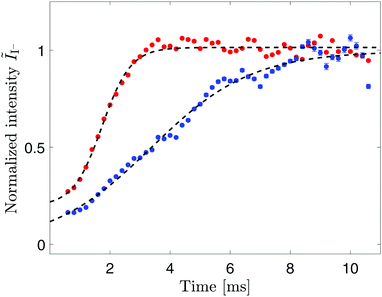Fig. 3 Thermalization of I− ions in the ion trap through collision with water vapor. The normalized intensities (see eqn (1)) were referenced to the intensity at 10 ms trapping time. The solid points show the normalized intensities of detected I− as a function of trapping time obtained with mean pressures of pH2O = 2.7 × 10−6 mbar (red), and pH2O = 1.3 × 10−6 mbar (blue) equivalent to water vapor densities of [H2O] = 6.4 × 1010 cm−3 and [H2O] = 3.2 × 1010 cm−3 at 300 K, respectively. The black dashed lines show a fit with the model function in eqn (7).

The intensity of extracted I initially increases and then becomes constant after a time that clearly depends on the water density. This observation can be attributed to elastic ion–molecule collisions12 whereby the ensemble of trapped I thermalizes (cools) to the temperature (∼300 K) of the inlet water vapor. The cooled ensemble of ions are more centered in the ion trap than the initially trapped hot ions, which effectively leads to an increased efficiency for extraction and transport through the QMS. In this sense, the data shown in Fig. 3 can be understood as the effective development of the detection efficiency εI during the thermalization process. The observed collisional cooling can be well described as a logistic function of the form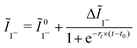(7)
where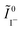is the initially observed intensity upon injection at the given vapor density, ΔĨI is the total change of observed intensity, rt describes an effective collisional cooling rate, and t0 is the midpoint of the cooling curve. Explicitly, for the data shown in Fig. 2 obtained with the higher water density (red points), we find rt = (2.10 ± 0.09) × 103 s−1 and t0 = (1.73 ± 0.03) ms.

For the present experimental investigation, the essential point from the data displayed in Fig. 2 is that it establishes a time scale for the cooling of an ion ensemble dependent on the water density. Thus, for example, at a density of [H2O] = 6.4 × 1010 cm−3 the cooling is established after ∼3 × t0 ∼5 ms.

### 4.2 I(H182O)− + H162O

The blue trace of Fig. 2 illustrates that when I(H182O) ions are trapped in the presence of H162O gas, two resulting ion products are observed, namely I and I(H162O) corresponding to collision-induced dissociation and exchange of H182O with H162O.

The corresponding temporal evolutions of the intensities of these product ions are displayed in Fig. 4. A strong increase of the intensity of I(H162O) as well as I is seen on a time scale that agrees well with the cooling time scales determined, as seen upon injection of bare I. The approximate thermalization phase is marked by the gray area. The intensity of the observed I(H182O) shows an initial small increase corresponding to collisional cooling and thereafter a decrease towards an apparently constant level at long times (>70 ms). To account for these observations, we consider the following reactions

 I(H182O)− + H162O → I(H182O)− + H162O (elastic collision) (8)
 → I(H162O)− + H182O (9)
 → I− + H182O + H162O (10)
The reaction in eqn (8) represents elastic collisions that tend to bring the I(H182O) ions in thermal equilibrium with the H162O gas. The reaction in eqn (9) represents the exchange of H182O with H162O. As an isolated reaction, eqn (9) requires a collisional energy above the difference in vibrational energy of the reaction and product systems. Our quantum chemical calculations reveal that this energy difference is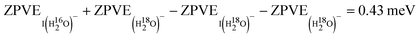(see Table 1). At thermal equilibrium at 300 K, this energy is available for approximately ∼99.84% of the I(H182O) + H162O collisions. Considering a possible inaccuracy of the calculated ZPVE of 0.1 meV, this fraction would change by only ±0.06%.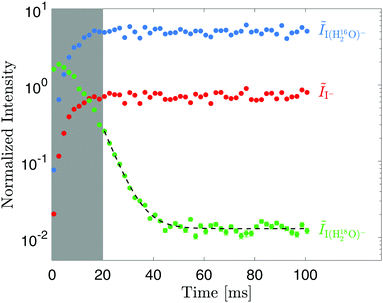Fig. 4 Observed normalized ion intensities (eqn (1)) as a function of trapping time after injection of I(H182O)− in the presence of water vapor at pH2O = 5.5 × 10−7 mbar ([H2O] = 1.3 × 1010 cm−3 at 300 K). The reference signal was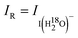at 10 ms. The dashed line shows a fit with the model given in eqn (12).

Finally, the reaction in eqn (10) is collision-induced dissociation of I(H182O) that requires collisional energy in excess of 0.26 eV.13 This reaction is therefore only relevant during the thermalization phase (gray area in Fig. 4) since such high collision energies are practically absent at 300 K.

Consistent with the reactions in eqn (9) and (10), the data displayed in Fig. 4 illustrate that most of the I(H162O) and I ions are formed during the injection and/or during the cooling period, where elevated reaction energies are available.

Beyond approximately 20 ms, when the thermalization to the gas temperature is progressed, reaction R3 becomes insignificant and the only possible loss of I(H182O) ions is through the exchange reaction in eqn (9). In this range, the evolution of the number of trapped I(H182O) ions can be approximated by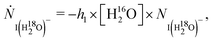(11)
where h1 is the reaction rate constant for the exchange reaction (eqn (9)), leading to a single exponential decay. This equation is, however, only approximate since, as indicated above, ∼0.2% of the trapped ions will not be able to react due to the reaction threshold of 0.43 meV. We interpret the almost constant level of I(H182O) intensity reached beyond 60–70 ms as a manifestation of this effect. To quantitatively model the data, we represent the observed decay of I(H182O) intensity in the form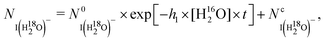(12)
where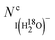represents ions not available for reaction in the exchange reaction (eqn (9)). It should be noted that the cold ions unavailable for the reaction in eqn (9) will eventually react since they are continuously subject to elastic collisions (eqn (8)). From a fit to the data in Fig. 4, we obtain a very significant reaction rate constant for the exchange reaction (eqn (9)) of h1 = (1.3 ± 0.1) × 10−8 cm3 s−1.

In summary, the results shown in Fig. 4 emphasize that even trace amounts of water in the inlet gas can have significant effect on the I(H182O)via the exchange reaction (eqn (9)).

### 4.3 I(H182O)− + 16O2

As seen from the green trace in Fig. 2, only I and I(H162O) appear as product ions when I(H182O) reacts with an inlet of 16O2 into the trap. Fig. 5 shows the corresponding time evolution of the ion product intensities. Both I and I(H162O) product ions increase far beyond the thermalization time (∼5 ms at a gas inlet pressure of 2.4 × 10−5 mbar), while the intensity of I(H182O) decreases after the initial increase during the cooling phase. It should be noted that the signal at mass 145 amu could in principle also originate from I18O; however, in our previous study of the I(H162O) + O2 reaction, IO products were not observed13 and hence, we also consider a contribution from I18O unlikely in the present I(H182O) + O2 experiment.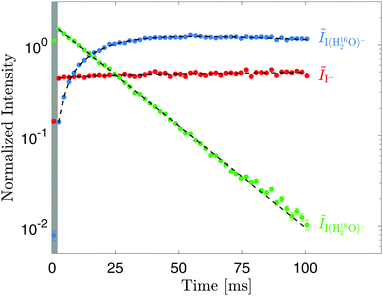Fig. 5 Observed ion intensities as a function of time after injection of I(H182O)− in the presence of oxygen with pO2 = 2.4 × 10−5 mbar ([O2] = 5.7 × 1011 cm−3). The reference signal wasat 10 ms. The dashed lines show a fit with the models given in eqn (15)–(17).

The fact that I(H162O) products are observed demonstrates that trace amounts of water are indeed present in the gas inlet.

To account for the observed intensities displayed in Fig. 5, the reactions in eqn (8) and (9) must be considered as well as the previously identified direct reaction of hydrated iodide and O2,13i.e.

 I(H162O)− + O2 → I− + neutrals (13)
 I(H182O)− + O2 → I− + neutrals. (14)
The corresponding rate equations for the trapped number of ion fragments are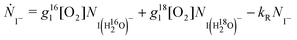(15)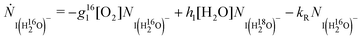(16)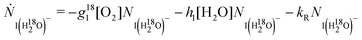(17)
where g161 and g181 are the reaction rate constants for the reactions in eqn (13) and (14), respectively, kR is a natural decay rate from the trap, and h1[H2O] is the effective rate of the exhange reaction (eqn (9)) due to the residual water in the gas inlet system. With g161, g181, h1[H2O], and kR as well as the initial ion product numbers as free parameters, eqn (15)–(17) can be fitted to the experimental data displayed in Fig. 5. The result of the fit is shown as dashed lines in Fig. 5 and the physically relevant reaction rate constants are summarized in Table 2 together with the value obtained for h1 from the data shown in Fig. 4.

Table 2 Experimentally determined reaction rate constants for hydrated iodine in collisions with water and oxygen (see also Fig. 4 and 5). The stated experimental error represents 68% confidence intervals from the fitting alone
Reaction Symbol Reaction rate constant (cm3 molecule−1 s−1)
I(H182O) + H162O → I(H182O) + H162O h 1 (1.3 ± 0.1) × 10−8
I(H162O) + O2 → I + neutrals g 161 (1.9 ± 0.2) × 10−12
I(H182O) + O2 → I + neutrals g 181 (3.2 ± 0.6) × 10−12

Additionally, the fit results in a value for the effective rate of h1 × [H2O] = (47.6 ± 2.0) s−1 for the exchange reaction (eqn (9)). Since the value of h1 was determined independently from the measurements with inlet of water vapor (h1 = 1.3 × 10−8 cm3 s−1) we can now explicitly estimate the actual water concentration to be [H2O] = 3.6 × 109 cm−3 or approximately 0.64% of the total inlet gas.

### 4.4 I(H182O)− + 16O3

When I(H182O) ions are trapped in the presence of both O2 and O3 as well as a trace amount of water, iodine oxide anions also appear as reaction products as seen in Fig. 2, which is consistent with the results obtained previously for I(H162O) + 16O3 collisions.13 Additionally, and perhaps surprisingly, a very clear signal of oxides, in particular the terminating IO3 is observed with an 18O atom originating from the water molecule initially hydrating the iodide anions. The corresponding time evolution of the detected product ion intensities is displayed in Fig. 6. The reactions potentially needed to account for the observed intensities are shown schematically in Fig. 7. In the ESI, we provide a table of reactions corresponding to this scheme and we also formulate explicitly the rate equations used to model the data in Fig. 6.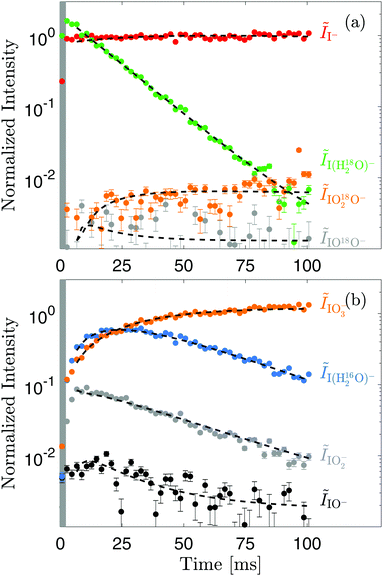Fig. 6 Observed ion intensities as a function of time after injection of I(H182O)− into the trap in the presence of oxygen, ozone, and trace amounts of water. (a) Intensities of iodide (I−) and ions that contain an 18O atom. (b) Intensities of ion products that contain only 16O atoms. The total pressure in the trap chamber was pO2 + pO3 = 2.0 × 10−5 mbar and the ozone fraction was 5.3%, i.e. [O2] = 4.5 × 1011 cm−3 and [O3] = 2.5 × 1010 cm−3 at 300 K. The reference signal wasat 10 ms. The dashed lines show a fit with the model indicated in Fig. 7 and formulated explicitly in the ESI.†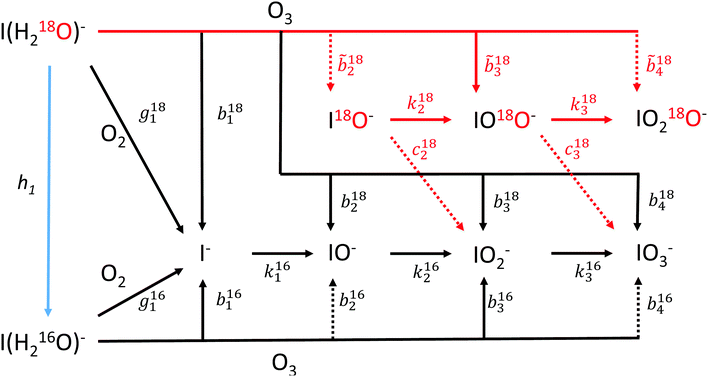Fig. 7 Schematic outline of the possible reactions taking place in the ion trap upon injection of I(H182O)− in the presence of inlet gas consisting of a mixture of O2 and O3 and with trace amounts of H2O. Black lines represent reactions where the 18O atom possibly has a minor mechanistic involvement, while red lines indicate reactions where the 18O must play an essential role for the reaction. The oxygen shown with black color represents 16O. The explicit reactions and the corresponding rate equations are given in the ESI.†

First, beyond the thermalization period, the initial growth of the I(H162O) intensity seen in Fig. 6(b) is evidence for the exchange of H182O with H162O on the hydrated iodide (reaction rate constant h1). Second, iodide I is formed with a slow growth rate (Fig. 6(a)) due to the reactions of both I(H182O) and I(H162O) with both O2 (reaction rate constants g181 and g161) and O3 (reaction rate constants b181 and b161). Third, from our previous studies of the reaction of bare I12 and I(H162O),13 it is well established that both of these ions react further with O3 to form IO3 either sequentially for I (reaction rate constant k161–3) or dominated by initial IO2 formation for I(H162O) (reaction rate constant b163) with weaker contributions from additional reactions (reaction rate constants b162 and b164). The data shown in Fig. 6(b) reflect this knowledge with the continuous growth of IO3 and the associated decline of IO and IO2.

The reactions of I(H182O) with O3 can both lead to I (reaction rate constant b181), and oxidized forms I16O1–3 where all associated oxygen atoms originate from the reacting O3, corresponding to a mechanistic picture where the H182O molecule does not play a significant role except being a carrier of energy and momentum.

We represent these processes with the reaction rate constants b182–4 in Fig. 7. Additionally, the presence of 18O in the hydrating water molecule of the iodide allows a further step in disentangling the reactions since oxidized fragments containing the 18O atoms can be identified. In the schematic diagram of Fig. 7, we represent the associated direct reactions from I(H182O) with the reaction rate constants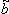182–3 and the subsequent oxidation reactions with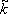182–3 for processes where the 18O atom remains on the ionic product, and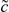182–3 for reactions where the the 18O atom is expelled.

From our previous measurements,12,13 the reaction rate constants involving only 16O (g161, k161–3, and b161–4) have been determined and in this work, a value for the exchange rate h1 has been established (see Fig. 4). Taking into account the uncertainties on these previous measurements and on the present data (Fig. 6), the processes indicated in Fig. 7 allow a good representation of the experimental results. An example of a representation (described below) is shown with the dashed lines in Fig. 6. A completely independent determination of the the additional 11 reaction rate constants (i.e. b181–4,182–4,182–3, and182–3) is evidently not meaningful with an unlimited fit to the data since various paths in the reaction scheme displayed in Fig. 7 are complementary.

Our main focus in this paper is to quantify the fraction of the I(H182O) + O163 that includes the 18O atom in the final reaction outcome. For this purpose, we artificially simplify the modeling of the reactions (Fig. 7) by fixing the values of h1, g161, g181, k161–3, b161–2, and b164 to the values estimated previously.13 Additionally, we set b181,4 = b161,4, and k182–3 = k162–3, and set explicitly182 =184 = 0 and182–3 = 0. With these restrictions, the data can then be fitted with b163, b182, b183, and183, as free parameters in addition to the initial ion numbers and the trace water concentration. With this choice, we effectively restrict the possible paths to IO182O and by keeping b163 as a free parameter, the uncertainties of the previous reaction rate constants effectively accumulate in its resulting absolute value from the fit. The fit obtained with these restrictions is displayed as the dashed lines in Fig. 6 and details of the fit restrictions and fit outcome are summarized in Table S1 (ESI). While the actual absolute numbers for the reaction rate constants resulting from the fit are only indicative due to the restrictions on the fit, the relative fraction of the product channels from the reactions I(H182O) + O163 that preserve the 18O atom in the ionic product can be directly evaluated as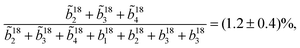(18)
where the given uncertainty reflects the combined error from the model fit.

## 5 Discussion

The presented experiments with isotope-substituted water attached to iodide, I(H182O), allowed us to determine the reaction rate constant (h1) for the exchange reaction (eqn (9)) where the water molecule attached to iodide is exchanged with an incoming water molecule at 300 K. The reaction barrier for the measured reaction is small (0.43 meV) and originates from the difference in zero-point vibrational energy for the two systems. The similar reaction with identical isotopes will be barrierless and likely its reaction rate constant will be similar or larger. Since the determined reaction rate constant for the water exchange reaction is rather large (h1 = 1.3 × 10−8 cm3 s−1), such water exchange processes could play a significant role in natural environments, where the ions are most often found with some degree of hydration, i.e. at low humidity a few hydrating water molecules are expected around iodide,13 while a very large hydration shell will surround the iodide at aqueous surfaces or in the bulk. In a previous study,13 we theoretically examined up to 6 hydrating water molecules, but the actual hydration shells for instance in bulk water are evidently larger. A particular effect of exchange reactions like the reaction in eqn (9) in natural environments could be an enhanced rate of thermalization, since an actual exchange of a particle is much more effective then elastic collisions (i.e. as the reaction in eqn (8)). We speculate that such enhanced cooling may be of importance for instance in connection with aerosol formation.20

The determined reaction rate constants for I(H2O) + O2 collisions, i.e. g161 and g181, are small (see Table 2) but could still be of significance in natural environments, since there the concentration of O2 is generally high, although, as mentioned above, naturally occurring hydrated iodide is likely found with more than one water molecule associated. In this context, it is also worth noting that hydrated ions are not (to the best of our knowledge) observed in ionic mass spectra obtained in field measurements.

It is a remarkable finding in this study that the reaction rate constants for the two hydrated systems, I(H162O) and I(H182O), with molecular oxygen (O2) differ by a factor of almost two (g181/g161 = 1.7 ± 0.3) and that the heavier system is associated with the larger reaction rate constant. This may be evidence that the heavier isotope has a stabilizing effect on a formed collision complex (I–H2O–O2), hence allowing more time for the dissociative break-up to occur; however, clearly more experiments and quantum chemistry calculations are needed to address this hypothesis.

A major result of this paper is the finding that the oxygen atom associated with the water molecule initially hydrating the iodide anion is found in approximately 1.2% of the resulting iodine oxides (mainly IO3) (see Fig. 6). In an intuitive picture of the collision where an O3 molecule approaches the I(H2O) anion, this may not be expected since the oxygen atom associated with the water molecule is far from the iodine atom, i.e. behind an I–H hydrogen bond, and a likely scenario for the reaction seems to be that the water molecule is simply facilitating the reaction by carrying energy and momentum away from a forming collision complex. As the present experiment reveals (Fig. 6), for at least 1.2% of the collisions the situation is much more complex and a much more involved reaction mechanism seems to be at play where significant reorganization of the molecular bonds takes place. Also in this case, both further dynamically oriented experimental investigations and quantum chemistry calculations would be needed to unravel the underlying mechanism behind this observation.

Finally, it should be emphasized that the involvement of the solvent water molecule has been quantified in the present study explicitly for the I(H182O) + O163 system. For naturally occurring I(H162O) + O163 collisions, the fraction of reactions where the oxygen from the water molecule will be found in the final IO3 product may evidently differ from the value determined here ((1.2 ± 0.4)%), but the qualitative result that the water molecule plays an active role in the reaction seems very likely to hold also for this reaction system.

## 6 Conclusion

With the present study of isolated ion–molecule collisions in an ion trap, we have highlighted the significance of the hydrating water molecules for the reactivity of iodine anions under humid or aqueous conditions that prevail, for example, where the atmosphere interfaces with the ocean, snow, or ice.

Previously, we have established that one hydrating water molecule can strongly modify the reaction paths and absolute reaction rate constants compared to the bare anion.12,13 Here, using isotope-substituted water molecules to hydrate the reacting iodide anion, we have explicitly found evidence for a deep mechanistic involvement of the water molecules in a non-negligible fraction (∼1.2%) of the anion collisions with ozone at 300 K.

We foresee to make similar experimental investigations also for other halogen anions (in particular bromide) and for anions with more associated water.

## Conflicts of interest

There are no conflicts to declare.

## Acknowledgements

We acknowledge support from the Danish Council for Independent Research and the Aarhus University Research Foundation (AUFF). J. E. thanks the Villum Foundation for financial support, and the Danish e-Infrastructure Cooperation (DeiC) for computational resources. We wish to thank Søren Baarsgaard Hansen, Aarhus University Hospital, for providing recycled samples of H182O.

## References

1. L. J. Carpenter, Chem. Rev., 2003, 103, 4953–4962 CrossRef CAS PubMed.
2. A. Saiz-Lopez, J. M. C. Plane, A. R. Baker, L. J. Carpenter, R. von Glasow, J. C. Gomez Martin, G. McFiggans and R. W. Saunders, Chem. Rev., 2012, 112, 1773–1804 CrossRef CAS PubMed.
3. W. R. Simpson, S. S. Brown, A. Saiz-Lopez, J. A. Thornton and R. v. Glasow, Chem. Rev., 2015, 115, 4035–4062 CrossRef CAS PubMed.
4. K. A. Read, A. S. Mahajan, L. J. Carpenter, M. J. Evans, B. V. E. Faria, D. E. Heard, J. R. Hopkins, J. D. Lee, S. J. Moller, A. C. Lewis, L. Mendes, J. B. McQuaid, H. Oetjen, A. Saiz-Lopez, M. J. Pilling and J. M. C. Plane, Nature, 2008, 453, 1232–1235 CrossRef CAS PubMed.
5. J. A. Garland and H. Curtis, J. Geophys. Res.: Oceans, 1981, 86, 3183–3186 CrossRef.
6. Y. Sakamoto, A. Yabushita, M. Kawasaki and S. Enami, J. Phys. Chem. A, 2009, 113, 7707–7713 CrossRef CAS PubMed.
7. E. A. Pillar, M. I. Guzman and J. M. Rodriguez, Environ. Sci. Technol., 2013, 47, 10971–10979 CrossRef CAS PubMed.
8. C. Moreno, M.-T. Baeza-Romero, M. Sanz, S. Gálvez, V. López Arza, J. C. Ianni and E. Espíldora, Phys. Chem. Chem. Phys., 2020, 22, 5625–5637 RSC.
9. C. G. Moreno, O. Gálvez, V. L.-A. Moreno, E. M. Espildora-Garca and M. T. Baeza-Romero, Phys. Chem. Chem. Phys., 2018, 20, 27571–27584 RSC.
10. C. Moreno and M. T. Baeza-Romero, Phys. Chem. Chem. Phys., 2019, 21, 19835–19856 RSC.
11. Q. Liu, L. M. Schurter, C. E. Muller, S. Aloisio, J. S. Francisco and D. W. Margerum, Inorg. Chem., 2001, 40, 4436–4442 CrossRef CAS PubMed.
12. R. Teiwes, J. Elm, K. Handrup, E. P. Jensen, M. Bilde and H. B. Pedersen, Phys. Chem. Chem. Phys., 2018, 20, 28606–28615 RSC.
13. R. Teiwes, J. Elm, M. Bilde and H. B. Pedersen, Phys. Chem. Chem. Phys., 2019, 21, 17546–17554 RSC.
14. O. Gálvez, M. Teresa Baeza-Romero, M. Sanz and L. F. Pacios, Phys. Chem. Chem. Phys., 2016, 18, 7651–7660 RSC.
15. M. J. Frisch, G. W. Trucks, H. B. Schlegel, G. E. Scuseria, M. A. Robb, J. R. Cheeseman, G. Scalmani, V. Barone, G. A. Petersson, H. Nakatsuji, et al., Gaussian 16, Revision A.03, Gaussian, Inc., Wallingford, CT, 2016 Search PubMed.
16. J. Chai and H. Martin, Phys. Chem. Chem. Phys., 2008, 10, 6615–6620 RSC.
17. K. A. Peterson, D. Figgen, E. Goll, H. Stoll and M. Dolg, J. Chem. Phys., 2003, 119, 11113–11123 CrossRef CAS.
18. K. A. Peterson, B. C. Shepler, D. Figgen and H. Stoll, J. Phys. Chem. A, 2006, 110, 13877–13883 CrossRef CAS PubMed.
19. https://cccbdb.nist.gov/vibscalejust.asp .
20. M. Sipilä, N. Sarnela, T. Jokinen, H. Henschel, H. Junninen, J. Kontkanen, S. Richters, J. Kangasluoma, A. Franchin, O. Peräkylä, M. P. Rissanen, M. Ehn, H. Vehkamäki, T. Kurten, T. Berndt, T. Petäjä, D. Worsnop, D. Ceburnis, V.-M. Kerminen, M. Kulmala and C. O'Dowd, Nature, 2016, 537, 532–534 CrossRef PubMed.

### Footnote

Electronic supplementary information (ESI) available: Rate equations used for modeling parts of the presented data and a table with a summary of a fit to these data See DOI: 10.1039/d0cp03219k
 This journal is © the Owner Societies 2020